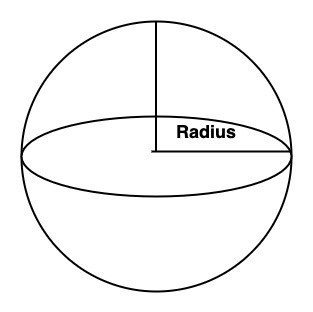# Swift Program to calculate the volume and area of Sphere

This tutorial will discuss how to write a Swift program to calculate the volume and area of the Sphere.

A sphere is a three-dimensional round solid shape or object. Or we can say a sphere is a shape that is defined in three axes: x-axis, y-axis, and z-axis. It does not hold any vertices or edges.## Volume of the Sphere

The amount of space occupied by a sphere in the three-dimensional plane is known as the volume of a sphere. For example, we want to fill a spherical ball with liquid, so using volume we can calculate the required amount of liquid.

### Formula

Following is the formula for volume −

Volume = 4πr3/3


Below is a demonstration of the same −

Input

Suppose our given input is −

Radius = 3


Output

The desired output would be −

Volume = 50


### Algorithm

Following is the algorithm −

• Step 1 − Declare a variable of double type to store the radius of the sphere.

var sRadius : Double = 4.0

• Step 2 − Declare another variable to store the volume of the sphere using the mathematical formula −

var sVolume = (4 * Double.pi * sRadius * sRadius * sRadius)/3

• Step 3 − Print the output.

### Example

The following program shows how to find the volume of the sphere.

import Foundation
import Glibc

var sRadius : Double = 4.0

// Calculating the volume of the sphere

print("Hence the volume of the sphere is:", sVolume)


### Output

Radius of the sphere is: 4.0
Hence the volume of the sphere is: 268.082573106329


Here in the above code, we calculate the volume of the sphere using the following code −

var sVolume = (4 * Double.pi * sRadius * sRadius * sRadius)/3


Display the volume of the sphere which is 268.082573106329 (Volume = (4 * 3.141592653589793 * 4.0 * 4.0 * 4.0)/3 = 268.082573106329).

## Area of the Sphere

The total space or region covered by the sphere in the three-dimensional plane is known as the area of the sphere.

### Formula

Following is the formula for the area of the sphere −

Area = 4πr2


Below is a demonstration of the same −

Input

Suppose our given input is −

Radius = 2


Output

The desired output would be −

Area = 50.26548245743669


### Algorithm

Following is the algorithm −

• Step 1 − Declare a variable of double type to store the radius of the sphere.

var sRadius : Double = 6.0

• Step 2 − Declare another variable to store the area of the sphere using the mathematical formula −

var sArea = 4 * Double.pi * sRadius * sRadius

• Step 3 − Print the output.

### Example

The following program shows how to find the area of a sphere.

import Foundation
import Glibc

var sRadius : Double = 6.0

// Calculating the area of the sphere

print("Hence the area of the sphere is:", sArea)


### Output

Radius of the sphere is: 6.0
Hence the area of the sphere is: 452.3893421169302


Here in the above code, we calculate the area of the sphere using the following code −

var sArea = 4 * Double.pi * sRadius * sRadius


Display the area of the sphere which is 452.3893421169302 (Volume = 4 * 3.141592653589793 * 6.0 * 6.0 = 452.3893421169302).